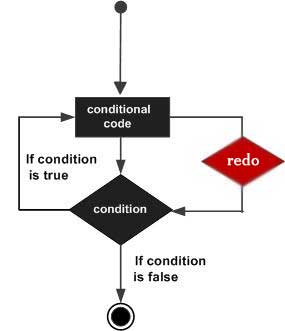# Perl redo Statement

The redo command restarts the loop block without evaluating the conditional again. You can provide a LABEL with redo statement where LABEL is the label for a loop. A redo statement can be used inside a nested loop where it will be applicable to the nearest loop if a LABEL is not specified.

If there is any continue block on the loop, then it will not be executed before evaluating the condition.

## Syntax

The syntax for a redo statement is as follows −

```redo [LABEL]
```

## Flow Diagram## Example

The following program shows the usage of redo statement −

```#/usr/local/bin/perl

\$a = 0;
while(\$a < 10) {
if( \$a == 5 ) {
\$a = \$a + 1;
redo;
}
print "Value of a = \$a\n";
} continue {
\$a = \$a + 1;
}
```

This would produce the following result −

```Value of a = 0
Value of a = 1
Value of a = 2
Value of a = 3
Value of a = 4
Value of a = 6
Value of a = 7
Value of a = 8
Value of a = 9
```
perl_loops.htm